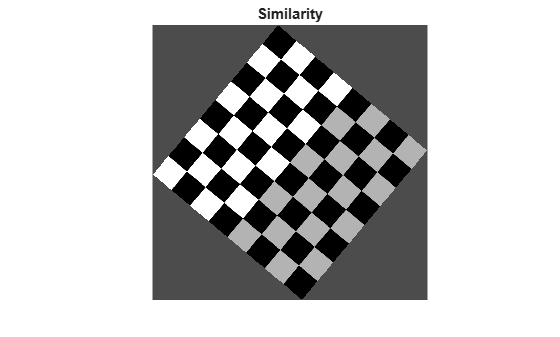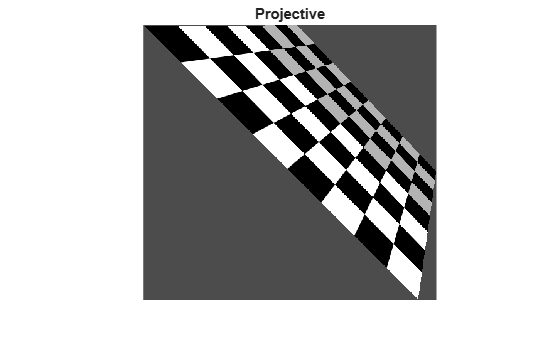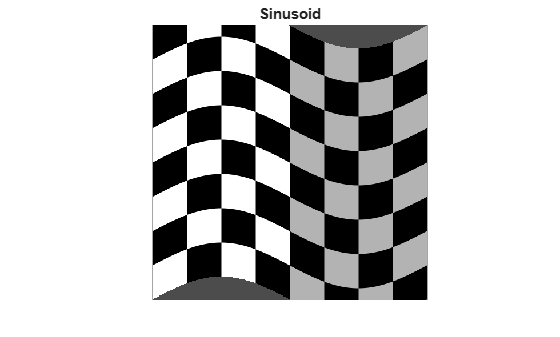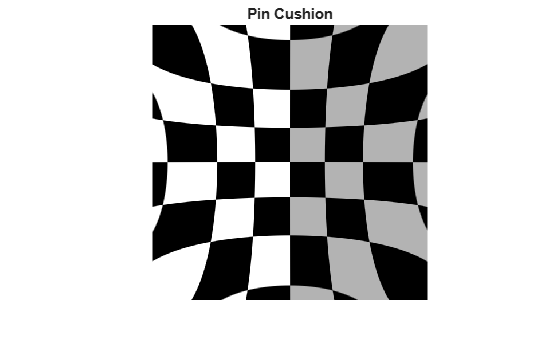# Create a Gallery of Transformed Images

This example shows many properties of geometric transformations by applying different transformations to a checkerboard image.

### Overview

A two-dimensional geometric transformation is a mapping that associates each point in a Euclidean plane with another point in a Euclidean plane. In these examples, the geometric transformation is defined by a rule that tells how to map the point with Cartesian coordinates (x,y) to another point with Cartesian coordinates (u,v). A checkerboard pattern is helpful in visualizing a coordinate grid in the plane of the input image and the type of distortion introduced by each transformation.

### Image 1: Create Checkerboard

`checkerboard` produces an image that has rectangular tiles and four unique corners, which makes it easy to see how the checkerboard image gets distorted by geometric transformations.

After you have run this example once, try changing the image `I` to your favorite image.

```sqsize = 60; I = checkerboard(sqsize,4,4); nrows = size(I,1); ncols = size(I,2); fill = 0.3; imshow(I) title('Original')```### Image 2: Apply Nonreflective Similarity to Checkerboard

Nonreflective similarity transformations may include a rotation, a scaling, and a translation. Shapes and angles are preserved. Parallel lines remain parallel. Straight lines remain straight.

For a nonreflective similarity,

`$\left[\begin{array}{c}u\phantom{\rule{0.5em}{0ex}}v\end{array}\right]=\left[\begin{array}{c}x\phantom{\rule{0.5em}{0ex}}y\phantom{\rule{0.5em}{0ex}}1\end{array}\right]\phantom{\rule{0.16666666666666666em}{0ex}}T$`

`T` is a 3-by-3 matrix that depends on 4 parameters.

```% Try varying these 4 parameters. scale = 1.2; % scale factor angle = 40*pi/180; % rotation angle tx = 0; % x translation ty = 0; % y translation sc = scale*cos(angle); ss = scale*sin(angle); T = [ sc -ss 0; ss sc 0; tx ty 1];```

Since nonreflective similarities are a subset of affine transformations, create an `affine2d` object using:

```t_nonsim = affine2d(T); I_nonreflective_similarity = imwarp(I,t_nonsim,'FillValues',fill); imshow(I_nonreflective_similarity); title('Nonreflective Similarity')```If you change either `tx` or `ty` to a non-zero value, you will notice that it has no effect on the output image. If you want to see the coordinates that correspond to your transformation, including the translation, include spatial referencing information:

```[I_nonreflective_similarity,RI] = imwarp(I,t_nonsim,'FillValues',fill); imshow(I_nonreflective_similarity,RI) axis on title('Nonreflective Similarity (Spatially Referenced)')```Notice that passing the output spatial referencing object `RI` from `imwarp` reveals the translation. To specify what part of the output image you want to see, use the 'OutputView' name-value pair in the `imwarp` function.

### Image 3: Apply Similarity to Checkerboard

In a similarity transformation, similar triangles map to similar triangles. Nonreflective similarity transformations are a subset of similarity transformations.

For a similarity, the equation is the same as for a nonreflective similarity:

`$\left[\begin{array}{c}u\phantom{\rule{0.5em}{0ex}}v\end{array}\right]=\left[\begin{array}{c}x\phantom{\rule{0.5em}{0ex}}y\phantom{\rule{0.5em}{0ex}}1\end{array}\right]\phantom{\rule{0.16666666666666666em}{0ex}}T$`

`T` is a 3-by-3 matrix that depends on 4 parameters plus an optional reflection.

```% Try varying these parameters. scale = 1.5; % scale factor angle = 10*pi/180; % rotation angle tx = 0; % x translation ty = 0; % y translation a = -1; % -1 -> reflection, 1 -> no reflection sc = scale*cos(angle); ss = scale*sin(angle); T = [ sc -ss 0; a*ss a*sc 0; tx ty 1];```

Since similarities are a subset of affine transformations, create an `affine2d` object using:

`t_sim = affine2d(T);`

As in the translation example above, retrieve the output spatial referencing object `RI` from the `imwarp` function, and pass `RI` to `imshow` to reveal the reflection.

```[I_similarity,RI] = imwarp(I,t_sim,'FillValues',fill); imshow(I_similarity,RI) axis on title('Similarity')```### Image 4: Apply Affine Transformation to Checkerboard

In an affine transformation, the x and y dimensions can be scaled or sheared independently and there may be a translation, a reflection, and/or a rotation. Parallel lines remain parallel. Straight lines remain straight. Similarities are a subset of affine transformations.

For an affine transformation, the equation is the same as for a similarity and nonreflective similarity:

`$\left[\begin{array}{c}u\phantom{\rule{0.5em}{0ex}}v\end{array}\right]=\left[\begin{array}{c}x\phantom{\rule{0.5em}{0ex}}y\phantom{\rule{0.5em}{0ex}}1\end{array}\right]\phantom{\rule{0.16666666666666666em}{0ex}}T$`

`T` is 3-by-3 matrix, where all six elements of the first and second columns can be different. The third column must be [0;0;1].

```% Try varying the definition of T. T = [1 0.3 0; 1 1 0; 0 0 1]; t_aff = affine2d(T); I_affine = imwarp(I,t_aff,'FillValues',fill); imshow(I_affine) title('Affine')```### Image 5: Apply Projective Transformation to Checkerboard

In a projective transformation, quadrilaterals map to quadrilaterals. Straight lines remain straight but parallel lines do not necessarily remain parallel. Affine transformations are a subset of projective transformations.

For a projective transformation:

`$\left[\phantom{\rule{0.16666666666666666em}{0ex}}\begin{array}{c}up\phantom{\rule{0.5em}{0ex}}vp\phantom{\rule{0.5em}{0ex}}wp\end{array}\phantom{\rule{0.16666666666666666em}{0ex}}\right]=\left[\phantom{\rule{0.16666666666666666em}{0ex}}\begin{array}{c}x\phantom{\rule{0.5em}{0ex}}y\phantom{\rule{0.5em}{0ex}}w\end{array}\phantom{\rule{0.16666666666666666em}{0ex}}\right]\phantom{\rule{0.16666666666666666em}{0ex}}T$`

`$u=\frac{up}{wp}$`

`$v=\frac{vp}{wp}$`

T is a 3-by-3 matrix, where all nine elements can be different.

`$T=\left[\begin{array}{c}A\phantom{\rule{0.2777777777777778em}{0ex}}D\phantom{\rule{0.2777777777777778em}{0ex}}G\\ B\phantom{\rule{0.2777777777777778em}{0ex}}E\phantom{\rule{0.2777777777777778em}{0ex}}H\\ C\phantom{\rule{0.2777777777777778em}{0ex}}F\phantom{\rule{0.2777777777777778em}{0ex}}I\end{array}\right]$`

The above matrix equation is equivalent to these two expressions:

`$u=\frac{Ax+By+C}{Gx+Hy+I}$`

`$v=\frac{Dx+Ey+F}{Gx+Hy+I}$`

Try varying any of the nine elements of `T`.

```T = [1 0 0.002; 1 1 0.0002; 0 0 1 ]; t_proj = projective2d(T); I_projective = imwarp(I,t_proj,'FillValues',fill); imshow(I_projective) title('Projective')```### Image 6: Apply Piecewise Linear Transformation to Checkerboard

In a piecewise linear transformation, affine transformations are applied separately to regions of the image. In this example, the top-left, top-right, and bottom-left points of the checkerboard remain unchanged, but the triangular region at the lower-right of the image is stretched so that the bottom-right corner of the transformed image is 50% further to the right and 20% lower than the original coordinate.

```movingPoints = [0 0; 0 nrows; ncols 0; ncols nrows;]; fixedPoints = [0 0; 0 nrows; ncols 0; ncols*1.5 nrows*1.2]; t_piecewise_linear = fitgeotrans(movingPoints,fixedPoints,'pwl'); I_piecewise_linear = imwarp(I,t_piecewise_linear,'FillValues',fill); imshow(I_piecewise_linear) title('Piecewise Linear')```### Image 7: Apply Sinusoidal Transformation to Checkerboard

This example and the following two examples show how you can create an explicit mapping to associate each point in a regular grid (xi,yi) with a different point (ui,vi). This mapping is stored in a `geometricTranform2d` object, which used by `imwarp` to transform the image.

In this sinusoidal transformation, the x-coordinate of each pixel is unchanged. The y-coordinate of each row of pixels is shifted up or down following a sinusoidal pattern.

```a = ncols/12; % Try varying the amplitude of the sinusoid ifcn = @(xy) [xy(:,1), xy(:,2) + a*sin(2*pi*xy(:,1)/nrows)]; tform = geometricTransform2d(ifcn); I_sinusoid = imwarp(I,tform,'FillValues',fill); imshow(I_sinusoid); title('Sinusoid')```### Image 8: Apply Barrel Transformation to Checkerboard

Barrel distortion perturbs an image radially outward from its center. Distortion is greater farther from the center, resulting in convex sides.

First, define a function that maps pixel indices to distance from the center. Use the `meshgrid` function to create arrays of the x-coordinate and y-coordinate of each pixel, with the origin in the upper-left corner of the image.

`[xi,yi] = meshgrid(1:ncols,1:nrows);`

Shift the origin to the center of the image. Then, convert the Cartesian x- and y-coordinates to cylindrical angle (`theta`) and radius (`r`) coordinates using the `cart2pol` function. `r` changes linearly as distance from the center pixel increases.

```xt = xi - ncols/2; yt = yi - nrows/2; [theta,r] = cart2pol(xt,yt);```

Define the amplitude, `a`, of the cubic term. This parameter is adjustable. Then, add a cubic term to `r` so that `r` changes nonlinearly with distance from the center pixel.

```a = 1; % Try varying the amplitude of the cubic term. rmax = max(r(:)); s1 = r + r.^3*(a/rmax.^2);```

Convert back to the Cartesian coordinate system. Shift the origin back to the upper-right corner of the image.

```[ut,vt] = pol2cart(theta,s1); ui = ut + ncols/2; vi = vt + nrows/2;```

Store the mapping between (`xi`,`yi`) and (`ui`,`vi`) in a `geometricTranform2d` object. Use `imwarp` to transform the image according to the pixel mapping.

```ifcn = @(c) [ui(:) vi(:)]; tform = geometricTransform2d(ifcn); I_barrel = imwarp(I,tform,'FillValues',fill); imshow(I_barrel) title('Barrel')```### Image 9: Apply Pin Cushion Transformation to Checkerboard

Pin-cushion distortion is the inverse of barrel distortion because the cubic term has a negative amplitude. Distortion is still greater farther from the center but the distortion appears as concave sides.

You can begin with the same `theta` and `r` values as for the barrel transformation. Define a different amplitude, b, of the cubic term. This parameter is adjustable. Then, subtract a cubic term to `r` so that `r` changes nonlinearly with distance from the center pixel.

```b = 0.4; % Try varying the amplitude of the cubic term. s = r - r.^3*(b/rmax.^2);```

Convert back to the Cartesian coordinate system. Shift the origin back to the upper-right corner of the image.

```[ut,vt] = pol2cart(theta,s); ui = ut + ncols/2; vi = vt + nrows/2;```

Store the mapping between (`xi`,`yi`) and (`ui`,`vi`) in a `geometricTranform2d` object. Use `imwarp` to transform the image according to the pixel mapping.

```ifcn = @(c) [ui(:) vi(:)]; tform = geometricTransform2d(ifcn); I_pin = imwarp(I,tform,'FillValues',fill); imshow(I_pin) title('Pin Cushion')```### Summary: Display All Geometric Transformations of Checkerboard

```figure subplot(3,3,1),imshow(I),title('Original') subplot(3,3,2),imshow(I_nonreflective_similarity),title('Nonreflective Similarity') subplot(3,3,3),imshow(I_similarity),title('Similarity') subplot(3,3,4),imshow(I_affine),title('Affine') subplot(3,3,5),imshow(I_projective),title('Projective') subplot(3,3,6),imshow(I_piecewise_linear),title('Piecewise Linear') subplot(3,3,7),imshow(I_sinusoid),title('Sinusoid') subplot(3,3,8),imshow(I_barrel),title('Barrel') subplot(3,3,9),imshow(I_pin),title('Pin Cushion')```Note that `subplot` changes the scale of the images being displayed.